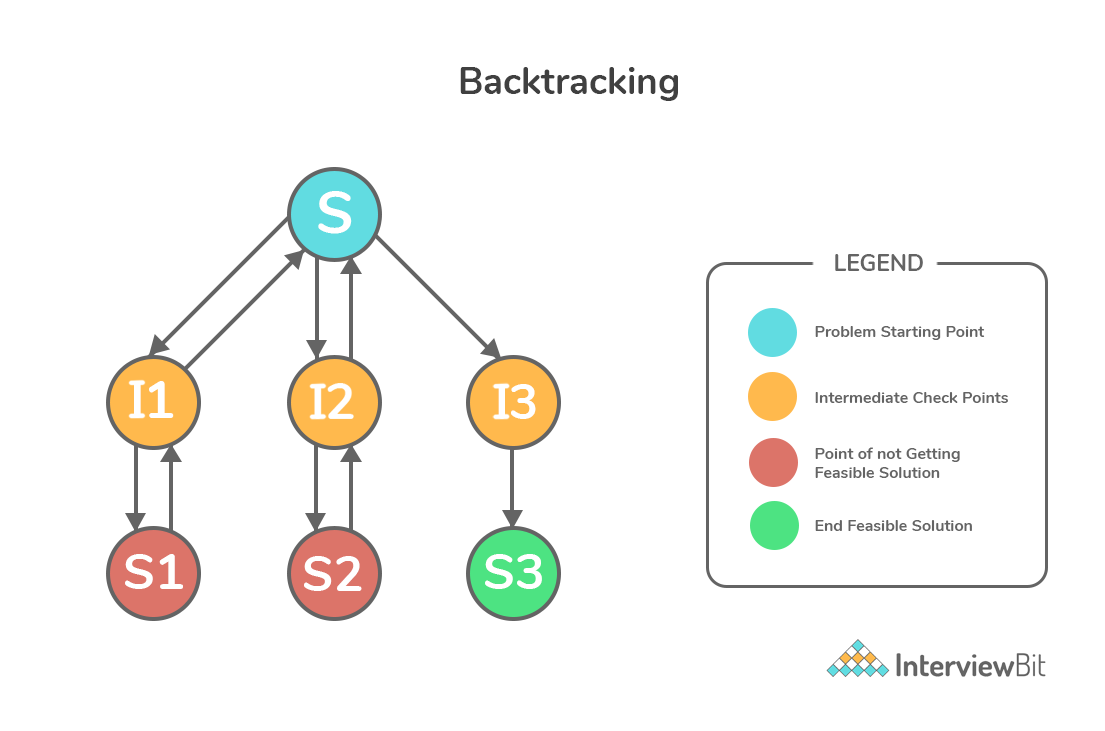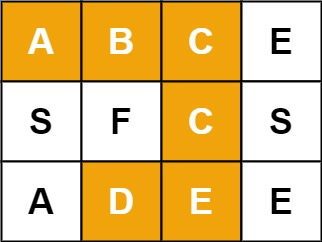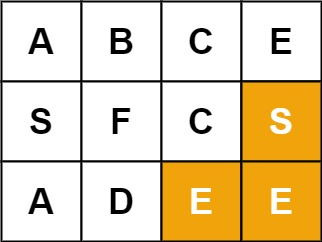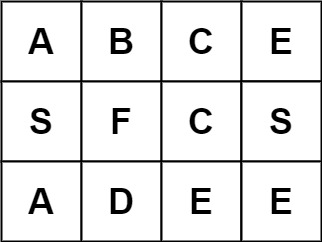### 回溯算法

#### 2 回溯是如何工作的？`S`是问题的出发点。

#### 3 回溯算法步骤

Step1 如果当前点是可行的解决方案，则返回success

Step2 否则，如果所有路径都已用尽（即当前点是终点），则返回失败，因为我们没有可行解

Step3 否则，如果当前点不是终点，则返回并探索其他点，然后重复上述步骤。

#### 4 回溯案例• 从根开始，你的选择是A和B。你选择A。
• 在A，你的选择是C和D。你选择C。
• C是坏的。回到A。
• 在A，您已经尝试了C，但失败了。试试D。
• D不好。回到A。
• 现在，你别无选择。回到根上。
• 在Root上，你已经试过A。试试B。
• 在B，你的选择是E和F。试试E。
• E是好的。

#### 5 回溯算法关键点

• 要结合递归遍历所有路径
• 每次回溯要能准确返回到检查点或状态点
• 尽可能今早判断出不满足的路径，也成为剪枝

#### 6 回溯算法注意事项

• 遍历所有可能情况，不能出现遗漏
• 回溯不能再重复之前遍历的情况，否则会陷入死循环
• 避免出现错误的可行解
• 回溯往往会结合递归，递归要有返回条件

#### 7 实际问题

##### 7.1 不含重复元素求子集

``````示例 1：

``````

``````class Solution:
def subsets(self, nums: List[int]) -> List[List[int]]:
n = len(nums)
res = []

def search(i, sol):
"""
:i: 代表回溯检查点
:sol: [0,i] 区间时解部分结构
"""
res.append(sol)
for j in range(i, n):
search(j + 1, sol + [nums[j]])

search(0, [])
return res
``````

##### 7.2 含重复元素求子集

``````示例 1：

``````

``````class Solution:
def subsetsWithDup(self, nums: List[int]) -> List[List[int]]:
n = len(nums)
res = []
nums.sort()
def search(i, sol):
if sol not in res:
res.append(sol)
for j in range(i, n):
search(j + 1, sol + [nums[j]])

search(0, [])
return res
``````

``````[4,4,4,1,4]
``````

``````[4,4,4,1]
[4,4,1,4]
``````
##### 7.3 单词搜索``````提示：

m == board.length
n = board[i].length
1 <= m, n <= 6
1 <= word.length <= 15
board 和 word 仅由大小写英文字母组成
``````

``````class Solution:
def exist(self, board: List[List[str]], word: str) -> bool:
if len(board) == 0:
return False
row_len, col_len, word_len = len(board), len(board), len(word)

def search(i, j, root):
"""
i,j is index in board
决策i,j是否放入root, 如果放入再进一步搜索
如果不能放入root，也就完全没必要进一步搜索
"""
k, tmp = len(root), root
if k == word_len:
return True
if i < 0 or j < 0 or i >= row_len or j >= col_len:
return False

if (i, j) not in root and board[i][j] == word[k]:
path = root + [(i, j)]
if (i - 1, j) not in root and search(i - 1, j, path):
return True
if (i + 1, j) not in root and search(i + 1, j, path):
return True
if (i, j - 1) not in root and search(i, j - 1, path):
return True
if (i, j + 1) not in root and search(i, j + 1, path):
return True
else:  # 剪枝
return False

for row in range(0, row_len):
for col in range(0, col_len):
if search(row, col, []):
return True
return False
``````